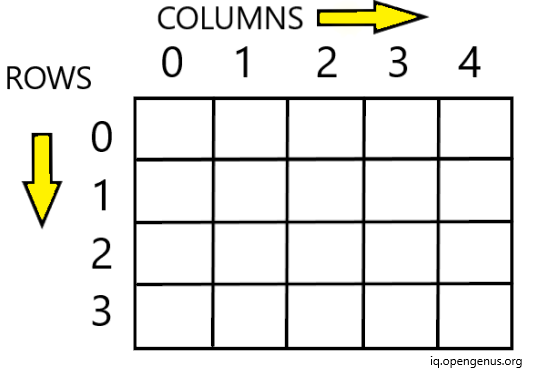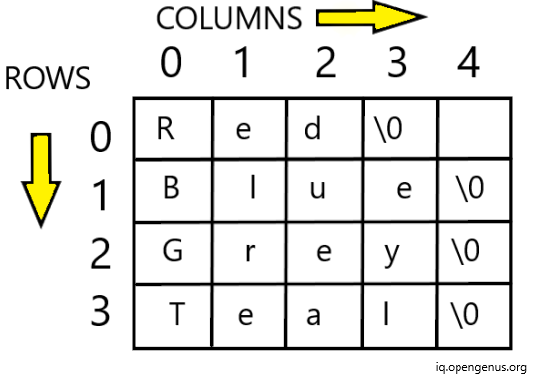×

Search anything:

# Different ways to initialize 2D array in C++

#### Software Engineering C++Arrays are the derived type in C++ that can hold values of same data type in contiguous memory allocation. In an array of size n, each value has an index number, starting from 0 till n-1.

We have explored different types to initialize 2D array in C++ like:

• Sized array initialization
• Skipping values initialization
• Unsized array initialization

### Types

Arrays are of two types:

1.One-Dimensional Arrays
2.Multi-Dimensional Arrays

## Two-Dimensional (2-D) Arrays

Multi-Dimensional Arrays comprise of elements that are themselves arrays. The simplest form of such arrays is a 2D array or Two-Dimensional Arrays. If an array has N rows and M columns then it will have NxM elements.

2-D Array Declaration is done as type array-name[rows][columns].

Example:

Below array arr has 4 Rows and 5 Columns having 4x5 Integer Elements.

``````int arr;
``````

Memory Representation of arr is as described below.The elements of arr are referred to as arr, arr and so on.

Similarly, a character array can be declared as:

``````char arr;
``````

It will always have one null character '\0' at the end of each row in last column.By specifying first index, the whole word can be accessed.

``````arr;
``````

Output:

``````Red
``````

# Initialization

### 1. Sized Array

C++ gives us the opportunity to initialize array at the time of declaration. For the arrays with specified size we initialize values as follows.

Example:
For a 2-Dimensional integer array, initialization can be done by putting values in curly braces "{" and "}". This list is called the value-list, a comma separated list of values of array elements. The data-type of these values should be same as data-type of the array (base type).

The following integer type array initializes a 2-D array of 5 rows and 2 columns, with total 5x2 = 10 elements.

``````int arr = {0,2,5,7,4,5,5,6,4,3};
``````

To enhance readability, we can do the same in other ways as follows:
Alternative Method 1:

``````int arr = {0,2,
5,7,
4,5,
5,6,
4,3
};
``````

Alternative Method-2:

``````int arr = {{0,2},{5,7},{4,5},{5,6},{4,3}};
``````

We can initialize the indivisual element in the array by specifying the row and column number.

Example:
Below code snippet initializes the element at 0 row index and 0 column index by 10.

``````arr=10;
``````

#### String arrays:

The Two-Dimensional, array of strings can be initialized as follows:

``````char str = {"Sunday","Monday","Tuesday","Wednesday","Thursday","Friday","Saturday"};
``````

Example:

Below is a program to initialize values of a 2-D integer array and print them.

``````#include<iostream>
using namespace std;
int main(){
int arr = {{2,2},
{5,8},
{4,3},
{26,22},
{4,32}
};
//To print the initialized values we use double for loop

for(int i=0;i<5;i++) // Outer for loop is to iterate over the Rows
{
for(int j=0;j<2;j++) //Inner for loop iterates over the columns in row equivalent to i
{
cout<<arr[i][j]<<" ";    //This statement prints the array element at arr[i][j]
}
cout<<"\n";   //This prints all elemnents of given row on one line and then moves to next line
}
return 0;
}
``````

Output:

``````2 2
5 8
4 3
26 22
4 32
``````

The time complexity for traversing a 2-D array of p rows and q columns will be O(p * q) since we use two loops. Outer loop to traverse rows and inner for columns.

## Skipping values:

In sized arrays if we skip some values while initialization, then those values are automatically taken as 0 by compiler.

Example:

``````#include <iostream>
using namespace std;

int main() {
//initializing array of 2 rows and 3 columns
int arr = {{ 2 },      // we skipped the values of second and third columns of first row
{ 0, 2 }  //We skipped the value of third column of second row
};

//Now we print the values of arr using for loop
for(int i=0;i<2;i++){
for(int j=0;j<3;j++){
cout<<arr[i][j]<<" ";
}
cout<<"\n";
}
return 0;
}
``````

Output:

``````2 0 0
0 2 0
``````

The values at index arr, arr in first row and at arr were replaced by 0 when no value was assigned to them during initialization of the two dimensional array.

Similarly, if we skip the initialization of whole row then all values will be automatically taken as 0.

Example:

``````#include <iostream>
using namespace std;

int main() {
//initializing array of 3 rows and 3 columns
int arr = {{ 2 },      // we skipped the values of second and third columns of first row
{ 0, 2 }  //We skipped the value of third column of second row
//We skipped all values of third row, it will be taken as {0,0,0}
};

//Now we print the values of arr using for loop
for(int i=0;i<3;i++){
for(int j=0;j<3;j++){
cout<<arr[i][j]<<" ";
}
cout<<"\n";
}
return 0;
}
``````

Output:

``````2 0 0
0 2 0
0 0 0
``````

## Unsized Array Initialization

We can also skip the size of the array during initialization. The C++ automatically creates an array that can hold all values. Such array is known as Unsized Array.

In 2-D arrays we can only skip the size of first index i.e. number of rows. The second index must be given i.e. the number of columns. The size of the first dimension is optional in C++ array initializations.

If we skip the size of first dimension in array then we must provide all the initializers(values) so that C++ can create the adequate memory for all values.

Example:

``````int square[]={{1,1},{2,4},{3,9}};
``````

Above code initializes the two dimensional unsized array named square by skipping the first index.

## Applications of 2-D Arrays:

Major Application of 2-D array revolves around the storage of large amount of data in tabular form i.e. in form of rows and columns. This representation is very similar to the relational databases that store data in form of relations (tables).

## Question

#### Which of these is the correct initialization of a two dimensional array?

int arr[][]={1,1};
int arr[]={2,3,3,3};
int arr[]={1,1,2,4,3,9};
int arr={2};
We can only skip the first index of two dimensional array that gives us the number of rows. Since it is a two dimensional array then it must have row and column index. Therefore, the correct answer is int arr[]={1,1,2,4,3,9};#### Srishti Guleria

Srishti Guleria has been a Technical Author, Software Developer, Intern at OpenGenus and a Computer Science student at Rajasthan Technical University, Kota

Different ways to initialize 2D array in C++